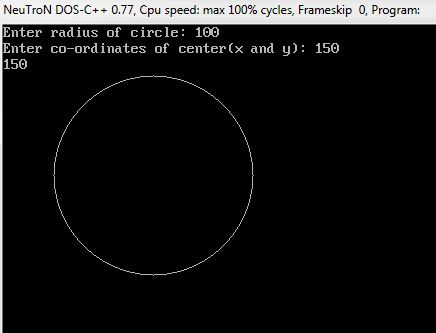# Midpoint Circle Algorithm in C and C++

Here you will get program for midpoint circle algorithm in C and C++. It is an algorithm used in computer graphics for drawing circle.

This program will work in Turbo C or Turbo C++ compiler as it uses graphics.h header file.

Change the path of BGI file inside initgraph() function according to your system to make this program run.

## Program for Midpoint Circle Algorithm in C++

Output### 19 thoughts on “Midpoint Circle Algorithm in C and C++”

1.Prasanta Kumar Sahoo

Nice
Thank you very much..

2.hello sir…
while i run this programme in turbo c++ 4.0 compiler, it takes the input of radius, x and y then shows…
BGI Error:Graphics not initialized (use ‘initgraph’)…

1.bro! read the lines before the program. it is directed “Change the path of BGI file inside initgraph() function according to your system to make this program run”.

2.change directory to bgi first,then save program again…also check linker to confirm graphics.h file is included or not

3.Crazy programmer

3.YOUR PROGRAM IS CORRECT BUT YOU SHOULD PUT A DELAY
FUNCTION IN WHILE LOOP.
OTHERWISE VERY GOOD FOR PROGRAMME.

4.5.Interesting example you’ve got here. I’ve tested already few code bits that using Bresenham algorithm, i have to say, your implementation is most ’round’ one 🙂

Little question, how would you fill the circle with your method?

Cheers,
Ev

6.Super easy program sir Thanks for great Help ……

7.what is 7 in putpixel(x0 + x, y0 + y, 7); pls explain.

1.7 IS COLOR CODE FOR WHITE COLOR

8.sorry i could’t understand the need of 7 in this program. why not another numbers

1.You also change your colour code with other number , like 5 is for pink ..
And as well as you can use colour name in Capital letter ” GREEN ” like this..

9.Thank you this worked perfectly for me

10.#include
#include
#include
#include
void main()
{
int gdriver=DETECT, gmode, error, x, y, r;
initgraph(&gdriver, &gmode, “c:\\turboc3\\bgi”);
cout<>r;
cout<>x>>y;
int x0=x;
int y0=y;
x = 0;
y = r;
int pk = 1-r;

while (x<y)
{
putpixel(x0 + x, y0 + y, 7);
putpixel(x0 + y, y0 + x, 7);
putpixel(x0 – y, y0 + x, 7);
putpixel(x0 – x, y0 + y, 7);
putpixel(x0 – x, y0 – y, 7);
putpixel(x0 – y, y0 – x, 7);
putpixel(x0 + y, y0 – x, 7);
putpixel(x0 + x, y0 – y, 7);
delay(10);

if (pk < 0)
{
x += 1;
pk = pk + 2*x + 1;
}
else
{
x += 1;
y -= 1;
pk = pk – 2*y + 2*x + 1;
}
}
getch();
closegraph();
}

11.draw a circle using midpoint circle algorithm then draw two lines cross sectioned with each other above the circle where section point is centre of the circle. How to solve this???

12.Sai Samanvitha SAI Hanumanthu

What is err?